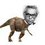# Let's see who really can count numbers

How many 5 digit positive integers are there such that the unit's place is 4 and it is divisible by 6?

Nice proofs and solutions are always welcome!Note by Nihar Mahajan
6 years, 2 months ago

This discussion board is a place to discuss our Daily Challenges and the math and science related to those challenges. Explanations are more than just a solution — they should explain the steps and thinking strategies that you used to obtain the solution. Comments should further the discussion of math and science.

When posting on Brilliant:

• Use the emojis to react to an explanation, whether you're congratulating a job well done , or just really confused .
• Ask specific questions about the challenge or the steps in somebody's explanation. Well-posed questions can add a lot to the discussion, but posting "I don't understand!" doesn't help anyone.
• Try to contribute something new to the discussion, whether it is an extension, generalization or other idea related to the challenge.

MarkdownAppears as
*italics* or _italics_ italics
**bold** or __bold__ bold
- bulleted- list
• bulleted
• list
1. numbered2. list
1. numbered
2. list
Note: you must add a full line of space before and after lists for them to show up correctly
paragraph 1paragraph 2

paragraph 1

paragraph 2

[example link](https://brilliant.org)example link
> This is a quote
This is a quote
    # I indented these lines
# 4 spaces, and now they show
# up as a code block.

print "hello world"
# I indented these lines
# 4 spaces, and now they show
# up as a code block.

print "hello world"
MathAppears as
Remember to wrap math in $$ ... $$ or $ ... $ to ensure proper formatting.
2 \times 3 $2 \times 3$
2^{34} $2^{34}$
a_{i-1} $a_{i-1}$
\frac{2}{3} $\frac{2}{3}$
\sqrt{2} $\sqrt{2}$
\sum_{i=1}^3 $\sum_{i=1}^3$
\sin \theta $\sin \theta$
\boxed{123} $\boxed{123}$

Sort by:

Fill the thousands, hundreds, and tens digits arbitrarily. Now there are exactly three ways to fill the ten-thousands digit. This easily gives $10^3 \cdot 3 = \boxed{3000}$ numbers.

- 6 years, 2 months ago

There are 15000 5 digit numbers that are divisible by 6.The first i.e the smallest one ending in 2.The unit digit of these numbers make a pattern,2,8,4,0,6,2,8,4,0,6,2....Hence 1 in every 5 consecutive numbers ends in 4.Hence answer to the problem is ,15,000/5=3000.

- 6 years, 2 months ago

Damn simple prob!Two second question!

- 6 years, 2 months ago

Nice solution!

- 6 years, 2 months ago

Thanx bud!

- 6 years, 2 months ago

Let the required $5$ digit number be $\overline{a_1a_2a_3a_44}$

Since the number is already divisible by $2$ we need to assure the divisibility of $3$

So , $\displaystyle\sum_{i = 1}^4 a_i \equiv -4 \equiv 2 \pmod{3}$

So , $\displaystyle\sum_{i =1}^4 a_i = 3k+2$ where $\begin{cases} 1 \leq k \leq 11 \\ 1 \leq a_1 \leq 9 \\ 0 \leq a_2,a_3,a_4 \leq 9 \end{cases}$

Indeed , finding solutions to this equation will fetch the required answer.

- 6 years, 2 months ago

No need to complicate it this much bud!

- 6 years, 2 months ago

But we must learn different methods.May be this method can help in some other problem.

- 6 years, 2 months ago

But if there are two different methods to solve a prob we must take the shorter solution.Donn'y u think?

- 6 years, 2 months ago

I know we must have the shorter one , but if the shorter method is not applicable to some other problem then what will you do? If we do not get a pattern in some other problem this method will not work.

- 6 years, 2 months ago

True!Let me tell you the method of solving this.Because $a_i\leq 9$,you can assume $a_1=9-x,a_2=9-y,a_3=9-z,a_4=9-p$.Notice here that $x,y,z,p$ are non-negative integers.Now you can consider every case and use stars and bars.But it would be very tedious.

- 6 years, 2 months ago

Yeah , thats a great idea. Let me see what happens next.

- 6 years, 2 months ago

Learn mthods that increase problem solving speed and increase accuracy, complicating things may take you down. :)

- 6 years, 2 months ago

Of course , a short and quick method is always preferred.But we must know different approaches to solve.May be the "short and quick" method does not work in some problems.In that case such different approaches may prove useful.Hence I prefer doing the same problem using different methods , rather that leaving it by solving it with the shortest method. :)

- 6 years, 2 months ago

It can also be seen as the number of four digit numbers that are divisible by 3, because the rest of the digits simply need to add upto a number that is one less than a multiple of 3. Under 1000 you have 333 such numbers and under a 10000 you have 3333 numbers divisible by 3. So we get 3000 by subtraction.

- 6 years, 2 months ago

30

- 6 years, 2 months ago

First term is 10014 and last term is 99984 (can easily be found by hit and trial method). These are the corresponding terms of an arithmetic progression with common difference = 30. An = a + (n-1)d, 99984 = 110014 + (n-1) 30, n = 3000

- 6 years, 2 months ago

The numbers are of the form 10k+4. We set 10k+4=6z or 5k+2=3z. We see that when k=-1 and z = -1 there is a solution note that when k= -1+3b and z = -1+5b. 5k+2= 5(-1+3b)+2=15b-3=3(5b-1)=3z. By euclidean algorithm there is only one solution in the interval where k= -2,-1,0 and z= -4,-3,-2,-1,0. eg one solution for (k,w) in this specified domain. Now we have 6z=6(-1+5b)=30b-6. All such numbers are of this form. Now 10000=<30b-6<=99999. 334<=b<=3333. Number of integers in the range = no of integers from 1 to 3333 - no of integers from 1 to 333 = 3333-333=3000

- 6 years, 2 months ago

we form a AP here : 10014, 10044, 10074............................................................99984 therefore no. of terms n= 99984-10014/30 +1 =3000

- 6 years, 2 months ago